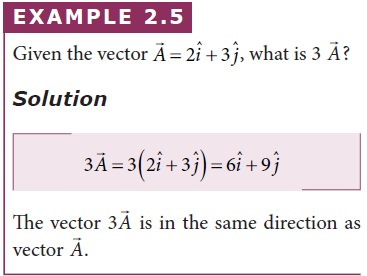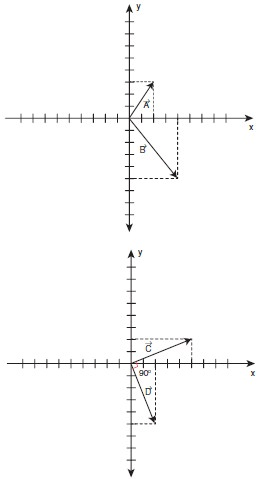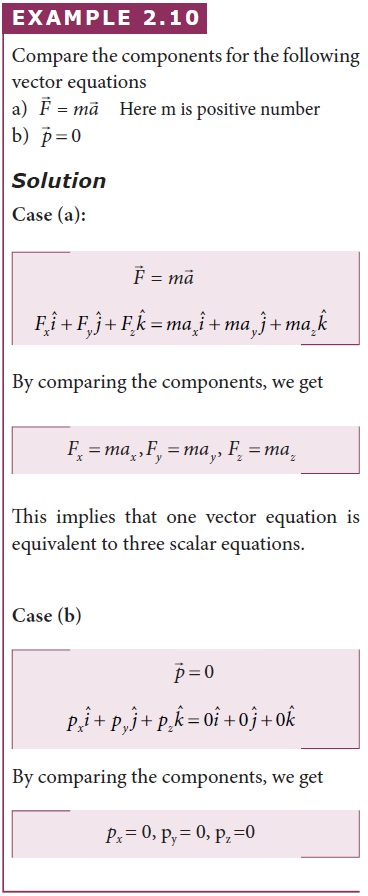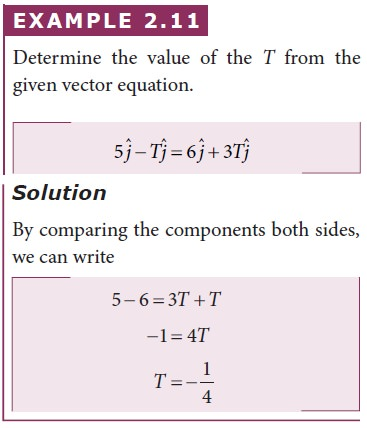Home | | Physics 11th std | Solved Example Problems for Multiplication of Vector by a Scalar

# Solved Example Problems for Multiplication of Vector by a Scalar

Physics : Kinematics : Multiplication of Vector by a Scalar

## Solved Example Problems for Multiplication Of Vector By A Scalar## Solved Example Problems for Scalar Product of Two Vectors## Solved Example Problems for The Vector Product of Two Vectors## Solved Example Problems for Properties of the components of vectorsStudy Material, Lecturing Notes, Assignment, Reference, Wiki description explanation, brief detail
11th Physics : UNIT 2 : Kinematics : Solved Example Problems for Multiplication of Vector by a Scalar |